Download the eSaral app and start learning from Kota's top IITians and doctors.

# Properties of Paramagnetic Substance Material - Class 12 Magnetism & Matters

The substances are weakly attracted by the magnetic field. In the non-uniform external magnetic field, paramagnetic substances move from week field region to a strong field region. A paramagnetic rod sets itself parallel to the field because the field is strongest near poles. properties of Paramagnetic Substance Material are as follows:   PROPERTIES OF PARAMAGNETIC SUBSTANCES MATERIAL
1. The substances which when placed in a magnetising field get feebly magnetised in a direction parallel to magnetising field are called paramagnetic.2. The substances are weakly attracted by the field so in non uniform field they have a tendency to move from strong to weak fields.
3. The substances are weakly attracted by the field so in non uniform field they have a tendency to move from strong to weak fields.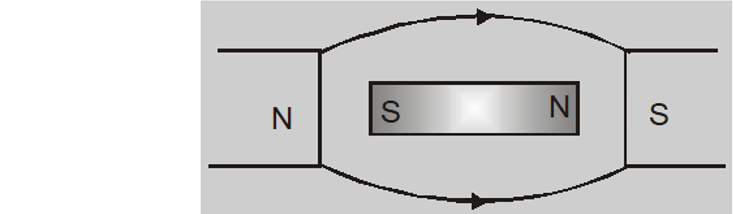4. A paramagnetic liquid in a U-tube ascends in the limb which is between the poles of magnet.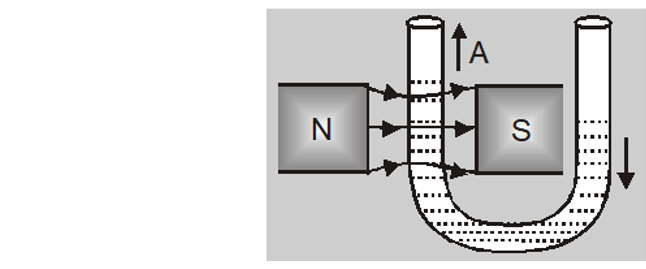5. Intensity of magnetisation I is very small, positive and directly proportional to magnetising field.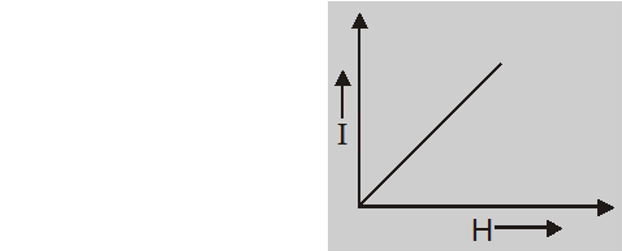6. Magnetic susceptibility $\chi_{\mathrm{m}}=\frac{\mathrm{I}}{\mathrm{H}}$ is small and positive $\left(\sim 10^{-3} \text { to } 10^{-5}\right)$
7. The relative permeability $\mu_{\mathrm{r}}=\frac{\mu}{\mu_{0}}$ is slightly greater than one. The field $B$ inside the material $B$ is greater than magnetising field H. They have a tendency to pull in the lines of force.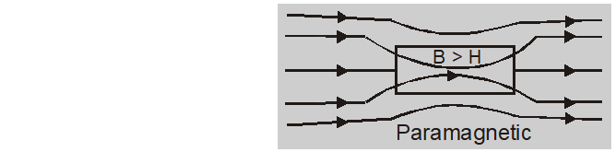8. Magnetic dipole moment (M) is small and parallel to magnetising field H.
9. The paramagnetic substances obey Curies law according to which magnetic susceptiblity is inversely proportional to its absolute temperature. $\quad \chi_{\mathrm{m}}=\frac{\mathrm{I}}{\mathrm{H}}=\frac{\mathrm{C}}{\mathrm{T}}$ temperature. $\quad \chi_{\mathrm{m}}=\frac{\mathrm{I}}{\mathrm{H}}=\frac{\mathrm{C}}{\mathrm{T}}$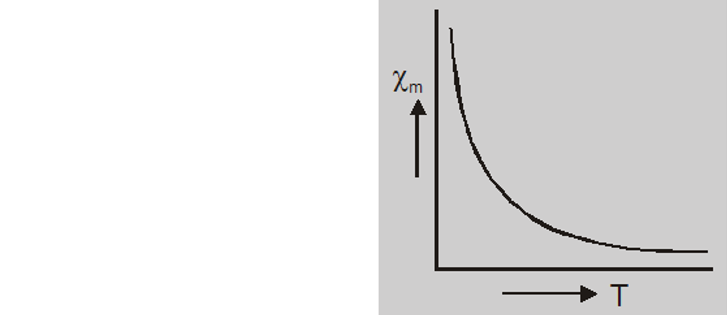10. Paramagnetism is due to partial alignment of randomly oriented atomic dipoles along the field.
11. The atoms of paramagnetic substances have permanent dipole moment i.e. unpaired spin.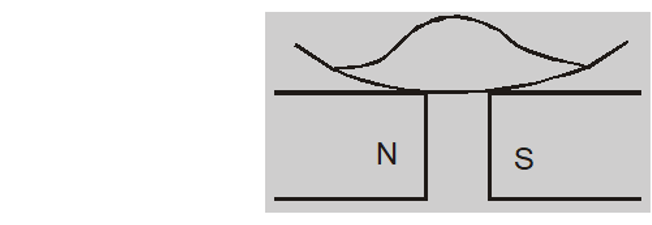12. Paramagnetism is exhibited by solids, liquids and gases.
13. If a paramagnetic liquid is placed in a watch glass placed on two pole pieces which are quite close to each other then liquids accumulates in the middle where field is strongest at poles.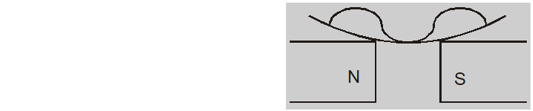14. Paramagnetic substances are $A \ell, N a, K, S b, P t, C u C \ell_{2}, M n,$ Cr, Sn , liquid oxygen etc.

Also Read: Properties of Paramagnetic & Diamagnetic Materials
About eSaral At eSaral we are offering a complete platform for IIT-JEE & NEET preparation. The main mission behind eSaral is to provide education to each and every student in India by eliminating the Geographic and Economic factors, as a nation’s progress and development depends on the availability of quality education to each and every one. With the blend of education & technology, eSaral team made the learning personalized & adaptive for everyone.